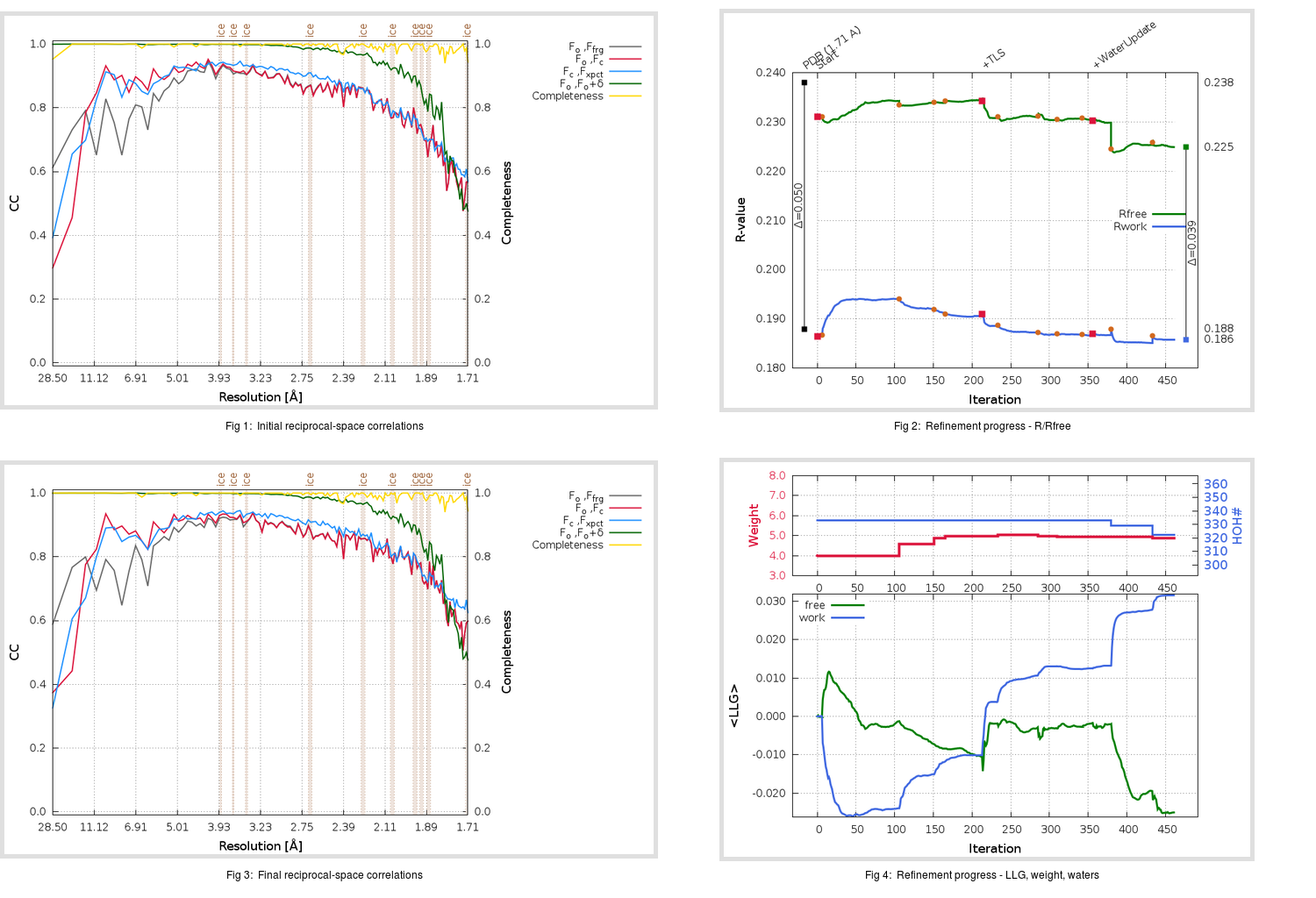Content:

## Deposited

` `
 Date deposited Date data collection Resolution R, Rfree 20200315 20200305 1.71 0.1850 0.2380

Molprobity (CCP4 7.0 version) summary:

```Ramachandran outliers =   0.33 %
favored =  99.01 %
Rotamer outliers      =   1.89 %
C-beta deviations     =     0
Clashscore            =   5.91
RMS(bonds)            =   0.0133
RMS(angles)           =   1.84
MolProbity score      =   1.53
Resolution            =   1.71
R-work                =   0.1850
R-free                =   0.2380
```

```Number of waters      =   333

<B> (all atoms) =   27.53 ( sd =   10.51 ) for       2731 non-hydrogen atoms
<B>   (protein) =   26.15 ( sd =    9.80 ) for       2370 non-hydrogen atoms
<B>     (water) =   36.53 ( sd =   10.65 ) for        333 non-hydrogen atoms
<B>    (others) =   38.23 ( sd =   14.16 ) for         28 non-hydrogen atoms

B min/max       (all non-hydrogen atoms) =   14.47 /   96.16
B min/max   (protein non-hydrogen atoms) =   14.47 /   83.85
B min/max     (water non-hydrogen atoms) =   16.95 /   96.16
B min/max     (other non-hydrogen atoms) =   25.04 /   61.97
```

## BUSTER (re-)refinement

` `

Molprobity (CCP4 7.0 version) summary:

```Ramachandran outliers =   0.33 %
favored =  98.68 %
Rotamer outliers      =   0.38 %
C-beta deviations     =     0
Clashscore            =   2.75
RMS(bonds)            =   0.0115
RMS(angles)           =   1.57
MolProbity score      =   1.06
Resolution            =   1.71
R-work                =   0.1859
R-free                =   0.2249
```

```Number of waters      =   322

<B> (all atoms) =   26.97 ( sd =    9.30 ) for       2720 non-hydrogen atoms
<B>   (protein) =   25.44 ( sd =    8.07 ) for       2370 non-hydrogen atoms
<B>     (water) =   37.39 ( sd =   10.38 ) for        322 non-hydrogen atoms
<B>    (others) =   37.92 ( sd =   15.03 ) for         28 non-hydrogen atoms

B min/max       (all non-hydrogen atoms) =   11.05 /   69.95
B min/max   (protein non-hydrogen atoms) =   11.05 /   69.95
B min/max     (water non-hydrogen atoms) =   12.60 /   68.27
B min/max     (other non-hydrogen atoms) =   22.90 /   52.60
```

Refinement progression:Results:

` `
 File Remark 5RFR_aB_refine.01_03_refine.pdb.gz exact refinement commands are in header 5RFR_aB_refine.01_03_refine.mtz.gz including original deposited data and several re-refinement map coefficients 5RFR_aB_refine.01_03_BUSTER_model.cif.gz including any non-standard compound restraints 5RFR_aB_refine.01_03_BUSTER_refln.cif.gz